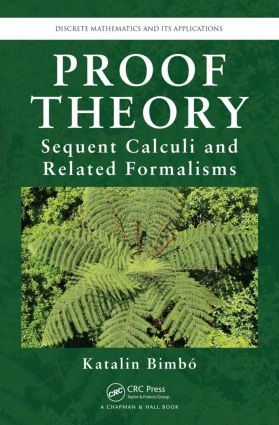Proof Theory

Sequent Calculi and Related Formalisms, 1st Edition

Chapman and Hall/CRC

386 pages | 13 B/W Illus.

Purchasing Options:\$ = USD
Hardback: 9781466564664
pub: 2014-08-20
SAVE ~\$22.00
\$110.00
\$88.00
x
eBook (VitalSource) : 9780429099694
pub: 2014-08-20
from \$28.98

FREE Standard Shipping!

Description

Although sequent calculi constitute an important category of proof systems, they are not as well known as axiomatic and natural deduction systems. Addressing this deficiency, Proof Theory: Sequent Calculi and Related Formalisms presents a comprehensive treatment of sequent calculi, including a wide range of variations. It focuses on sequent calculi for various non-classical logics, from intuitionistic logic to relevance logic, linear logic, and modal logic.

In the first chapters, the author emphasizes classical logic and a variety of different sequent calculi for classical and intuitionistic logics. She then presents other non-classical logics and meta-logical results, including decidability results obtained specifically using sequent calculus formalizations of logics.

The book is suitable for a wide audience and can be used in advanced undergraduate or graduate courses. Computer scientists will discover intriguing connections between sequent calculi and resolution as well as between sequent calculi and typed systems. Those interested in the constructive approach will find formalizations of intuitionistic logic and two calculi for linear logic. Mathematicians and philosophers will welcome the treatment of a range of variations on calculi for classical logic. Philosophical logicians will be interested in the calculi for relevance logics while linguists will appreciate the detailed presentation of Lambek calculi and their extensions.

Reviews

"Katalin Bimbo is one of the leading relevance logicians in the world today and indeed one of the leading non-classical logicians in general. Her book on proof theory takes readers through standard (classical) proof theory and beyond, including proof theory for some of the most important non-classical logics. The discussion is brilliantly executed. All graduate students interested in logic should study this book and all faculty too. I plan to use the book often."

—Jc Beall, Professor of Philosophy, University of Connecticut, and Professorial Fellow, Northern Institute of Philosophy, University of Aberdeen

Proofs and proof theory

Proofs of all kinds

Early history of proof theory in a nutshell

Proofs as calculations

Classical first-order logic

The sequent calculus LK

An axiom system for FOL

Equivalence of LK and K

Interpretations, soundness and completeness

Variants of the first sequent calculi

Intuitionistic logic and other modifications

Sequent calculi with multisets and sets

Sequent calculi with no structural rules

One-sided sequent calculi

Uniform sequent calculi

Disjunction property

Translations between classical and intuitionistic logics

Sequent calculi for non-classical logics

Associative Lambek calculus

Extensions of the associative Lambek calculus

Relevant implication and pure entailment

Non-distributive logic of relevant implication

Linear logic

Positive logic of relevant implication

Sequent calculi for modal logics

Merge calculi

Consecution calculi for non-classical logics

Non-associative Lambek calculus

Structurally free logics

More implicational relevance logics

Positive entailment logics

Calculi with multiple right-hand side

Display calculi and hypersequents

Display logics with star

Display logic for linear logic

Display logic for symmetric gaggles

Hypersequent calculi

Cut rules and cut theorems

Uniform cut theorem

Mix, multiple and single cuts

Constants and the cut

Display cut

Cut theorem via normal proofs

Cut theorem via interpretations

Analytic cut

Consequences of the cut theorem and uses of the cut rules

Some other proof systems

Natural deduction systems

Tableau systems

Resolution systems

Applications and applied calculi

Decidability

Sequent calculi for mathematical theories

Typed and labeled calculi

Appendix: Some supplementary concepts

Bibliography

Index